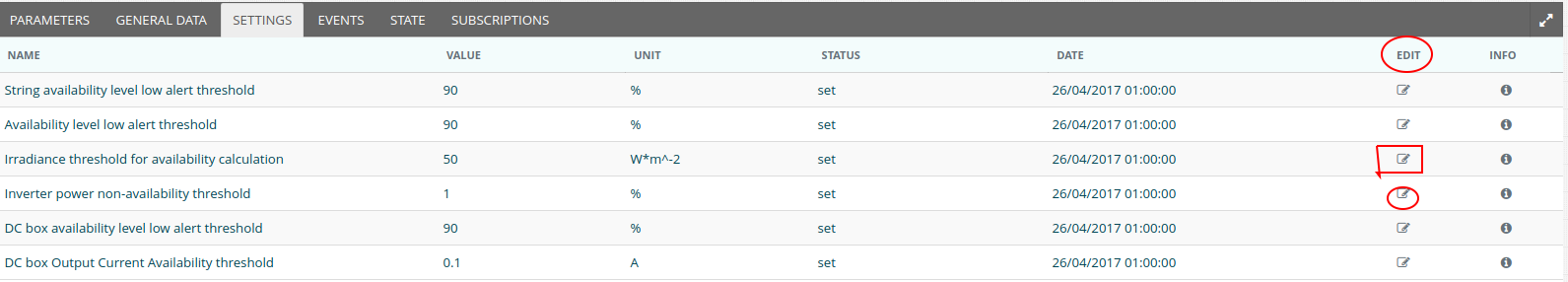The Availability (AL) parameter onsite level is calculated as weighted average from the corresponding availabilities on inverter level.

Availability calculation at inverter level

In each inverter, if the irradiance exceeds the "Irradiance threshold for availability calculation"  and if the inverter power/inverter nominal power is > "Inverter power non availability threshold", then inverter availability receives the value "1" for this quarter of hour.

You can find the "Irradiance threshold for availability calculation", "Inverter power non availability threshold" in the tab "Settings" as shown below. You can edit the Settings using the "Edit" icon.So if the inverter is producing normally, inverter Availability=1 (for the 15min interval)

If the inverter has stopped producing due to some error, inverter Availability=0 (for the 15min interval)

Availability calculation at site level

Let's suppose that in a site there are k inverters. The site level Availability formula is

15 min Availability=100%*(inv1_AL*inv1_nominalpower+...+invk_AL*invk_nominalpower)/(Site Nominal Power), as long as the condition "Irradiance PoA Average" > "Irradiance threshold for availability calculation" is satisfied.

Keep in mind that Site Nominal Power=inv1_nominalpower+...+invk_nominalpower

Availability calculation at site level example

Let's suppose a site with

2x1500kW inverter

2x1000kW inverters

For a given 15 min interval with Irradiance Average > Irradiance threshold one of the 1000kW inverters is not producing.

The Site Availability will be=100%*(1*1500+1*1500+1*1000+0*1000)/(5000)=80%

Daily/monthly/yearly Availability calculation

Daily Availability=average of the 15 min availability values for the given day

Monthly and yearly availability are calculated in the same manner.